# Example # 24.1: Find the magnetic field 5 cm from a long wire carrying 12 A....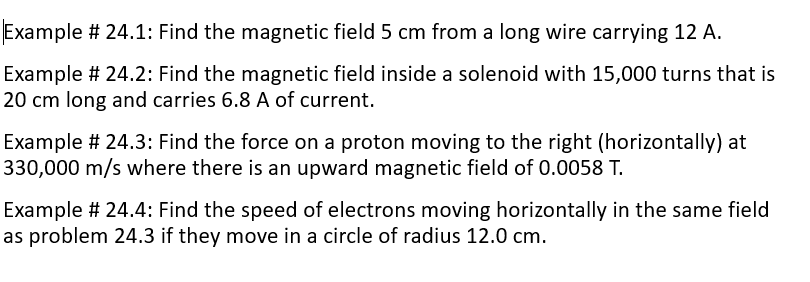Example # 24.1: Find the magnetic field 5 cm from a long wire carrying 12 A. Example # 24.2: Find the magnetic field inside a solenoid with 15,000 turns that is 20 cm long and carries 6.8 A of current. Example # 24.3: Find the force on a proton moving to the right (horizontally) at 330,000 m/s where there is an upward magnetic field of 0.0058 T. Example # 24.4: Find the speed of electrons moving horizontally in the same field as problem 24.3 if they move in a circle of radius 12.0 cm.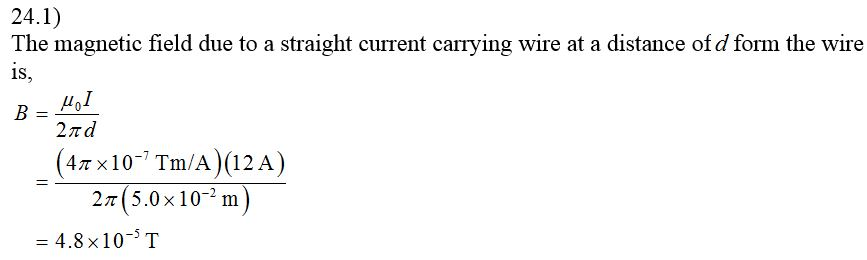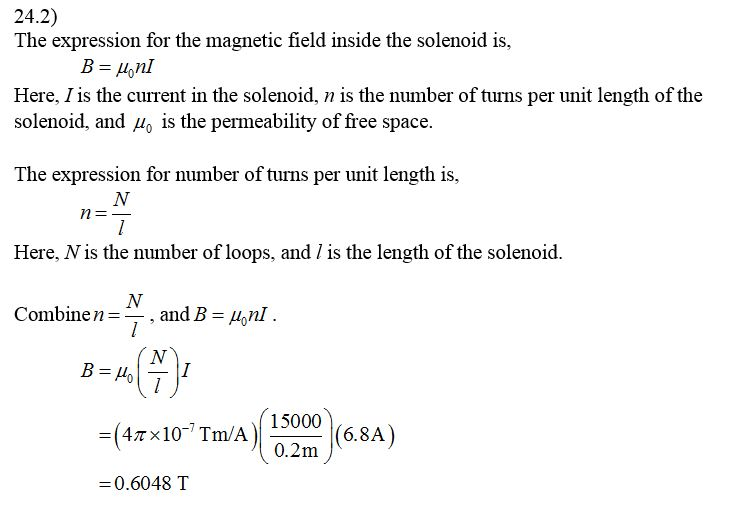#### Earn Coin

Coins can be redeemed for fabulous gifts.

Similar Homework Help Questions
• ### Example # 24.3: Find the force on a proton moving to the right (horizontally) at 330,000...

Example # 24.3: Find the force on a proton moving to the right (horizontally) at 330,000 m/s where there is an upward magnetic field of 0.0058 T. Example # 24.4: Find the speed of electrons moving horizontally in the same field as problem 24.3 if they move in a circle of radius 12.0 cm.

• ### Example # 24.3: Find the force on a proton moving to the right (horizontally) at 330,000...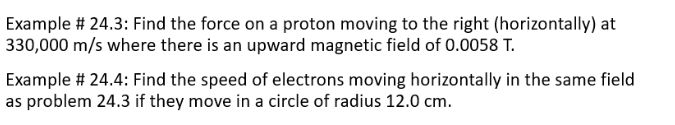Example # 24.3: Find the force on a proton moving to the right (horizontally) at 330,000 m/s where there is an upward magnetic field of 0.0058 T. Example # 24.4: Find the speed of electrons moving horizontally in the same field as problem 24.3 if they move in a circle of radius 12.0 cm.

• ### Example # 24.1: Find the magnetic field 5 cm from a long wire carrying 12 A.

Example # 24.1: Find the magnetic field 5 cm from a long wire carrying 12 A.

• ### The magnetic field 37.0 cm away from a long, straight wire carrying current 9.00 A is...

The magnetic field 37.0 cm away from a long, straight wire carrying current 9.00 A is 4860 µT. (a) At what distance is it 486 µT?   cm (b) At one instant, the two conductors in a long household extension cord carry equal 9.00-A currents in opposite directions. The two wires are 3.00 mm apart. Find the magnetic field 37.0 cm away from the middle of the straight cord, in the plane of the two wires.    How far is the...

• ### The magnetic field 43.0 cm away from a long, straight wire carrying current 9.00 A is...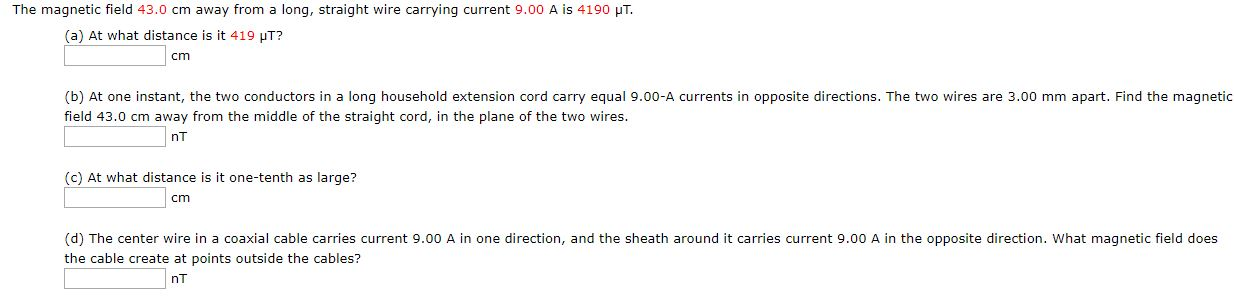The magnetic field 43.0 cm away from a long, straight wire carrying current 9.00 A is 4190 UT. (a) At what distance is it 419 HT? cm (b) At one instant, the two conductors in a long household extension cord carry equal 9.00-A currents in opposite directions. The two wires are 3.00 mm apart. Find the magnetic field 43.0 cm away from the middle of the straight cord, in the plane of the two wires. nt (c) At what distance...

• ### The magnetic field 37.0 cm away from a long, straight wire carrying current 7.00 A is...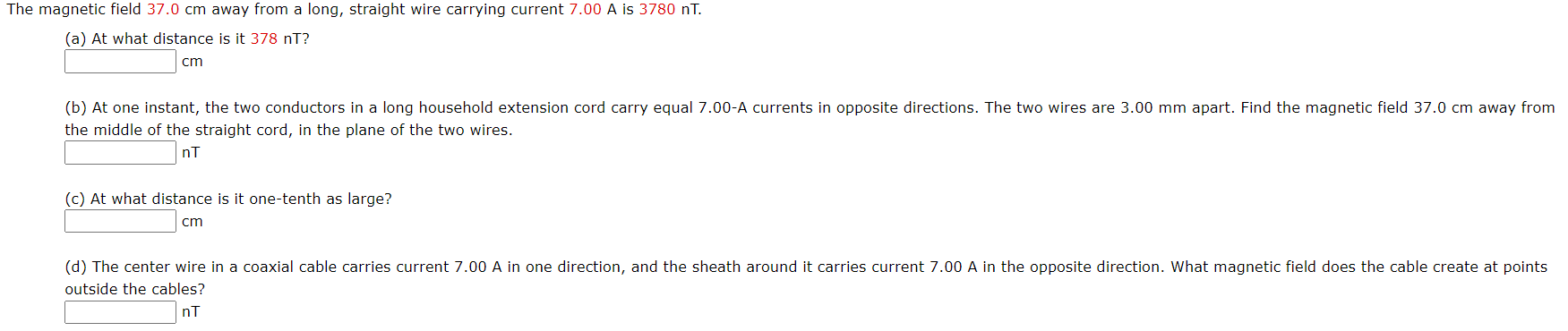The magnetic field 37.0 cm away from a long, straight wire carrying current 7.00 A is 3780 nT. (a) At what distance is it 378 nt? cm (b) At one instant, the two conductors in a long household extension cord carry equal 7.00-A currents in opposite directions. The two wires are 3.00 mm apart. Find the magnetic field 37.0 cm away from the middle of the straight cord, in the plane of the two wires. ni (c) At what distance...

• ### 5) A solenoid comprises 500,000 turns of wire and is 10 cm long and 1.0 cm...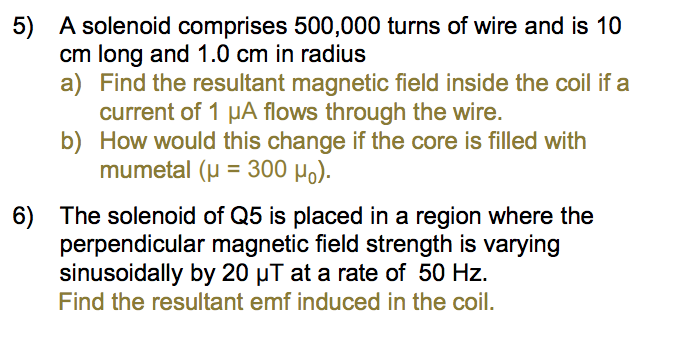5) A solenoid comprises 500,000 turns of wire and is 10 cm long and 1.0 cm in radius. a) Find the resultant magnetic field inside the coil if a current of 1 μA flows through the wire. b) How would this change if the core is filled with mumetal (μ = 300 μ0 ). 6) The solenoid of Q5 is placed in a region where the perpendicular magnetic field strength is varying sinusoidally by 20 μT at a rate of...

• ### 6) For the following diagrams: A) Draw the magnetic field for a wire carrying current. B)...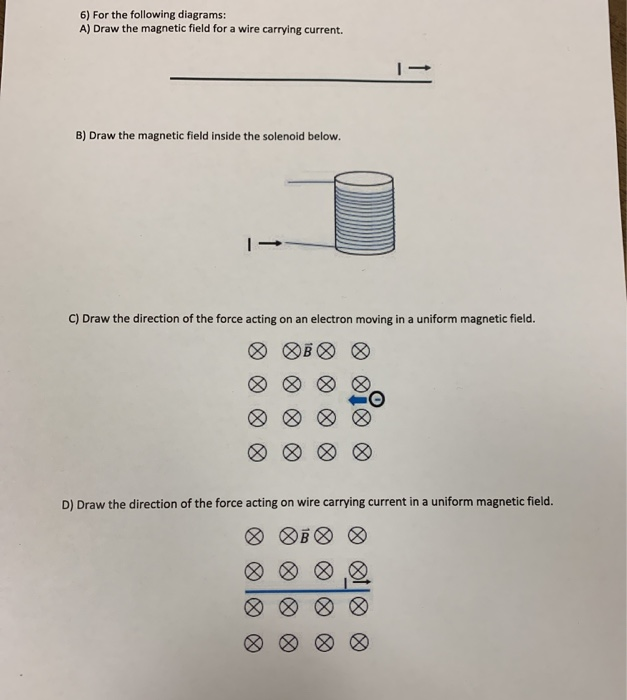6) For the following diagrams: A) Draw the magnetic field for a wire carrying current. B) Draw the magnetic field inside the solenoid below. C) Draw the direction of the force acting on an electron moving in a uniform magnetic field. D) Draw the direction of the force acting on wire carrying current in a uniform magnetic field. OB o olio o

• ### physics help he magnetic field 35.0 cm away from a long, straight wire carrying current 1.00...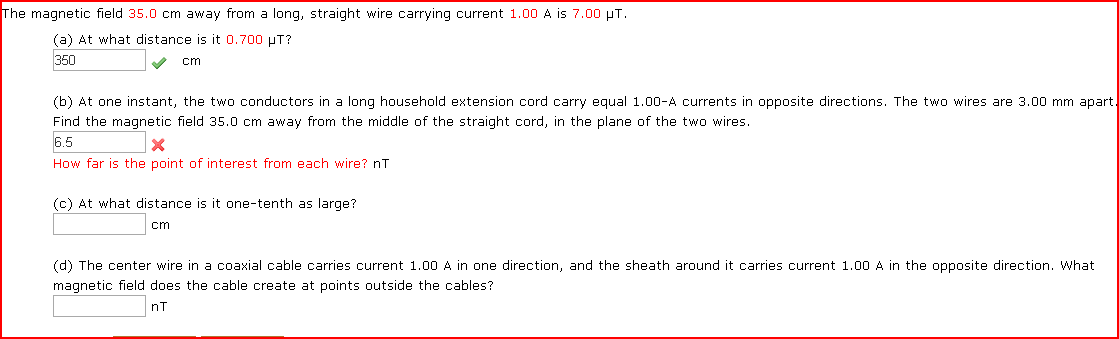physics help he magnetic field 35.0 cm away from a long, straight wire carrying current 1.00 A is 7.00 HT (a) At what distance is it 700 HT? 350 Cm (b) At one instant, the two conductors in a long household extension cord carry equal 1.00-A currents in opposite directions. The two wires are 3.00 mm apart Find the magnetic field 35.0 cm away from the middle of the straight cord, in the plane of the two wires. 6.5 How...

• ### Problem 5: A 5 cm long wire is in a uniform magnetic field of 4T, so...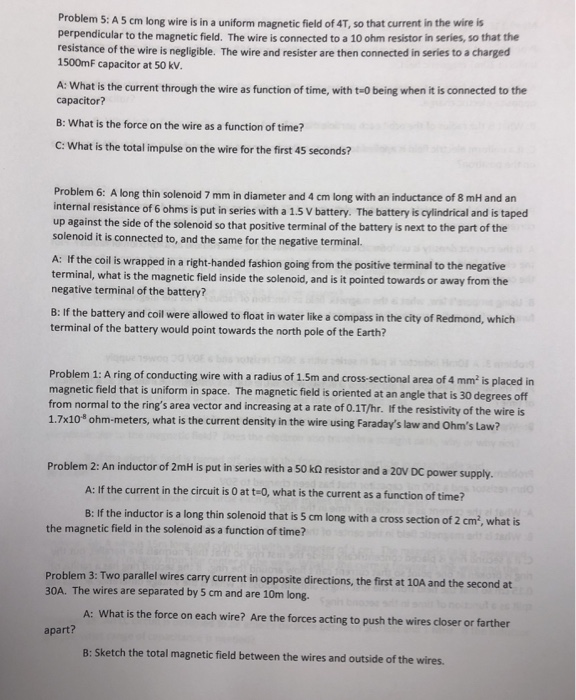Problem 5: A 5 cm long wire is in a uniform magnetic field of 4T, so that current in the wire is perpendicular to the magnetic field. The wire is connected to a 10 ohm resistor in series, so that the resistance of the wire is negligible. The wire and resister are then connected in series to a charged 1500mF capacitor at 50 kV. A: What is the current through the wire as function of time, with t-0 being when...